#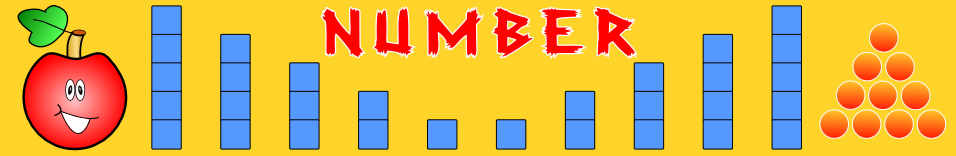There are 366 different Starters of The Day, many to choose from. You will find below some starters on the topic of Number. A lesson starter does not have to be on the same topic as the main part of the lesson or the topic of the previous lesson. It is often very useful to revise or explore other concepts by using a starter based on a totally different area of Mathematics.

Main Page

### Number Starters:Four different actions depending on the number which appears.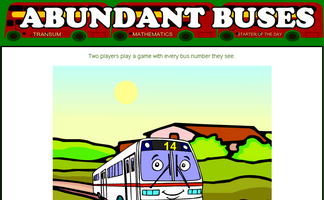A game based around the concept of factors and abundant numbers.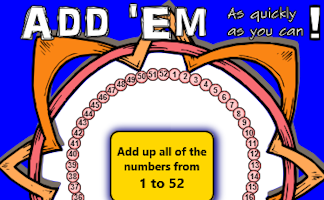Add up a sequence of consecutive numbers. Can you find a quick way to do it?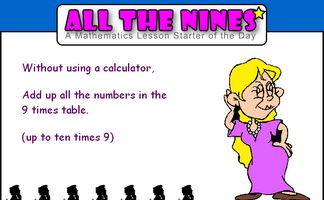Add up all the numbers in the nine times table.This activity requires students to memorise fifteen numbers in a three by five grid.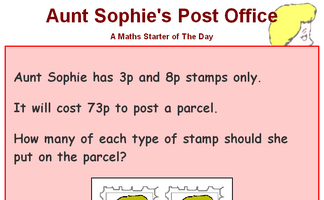Work out the number of stamps needed to post a parcel.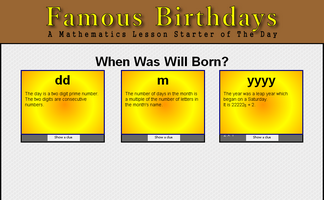Work out the date Will was born by answering some number questions.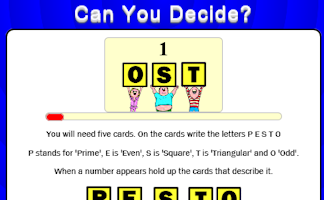Recognise odd, even, square, prime and triangular numbers.If all the bells ring together at noon, at what time will they next all ring together? This problem requires the use of LCM.How many palindromic numbers can you find?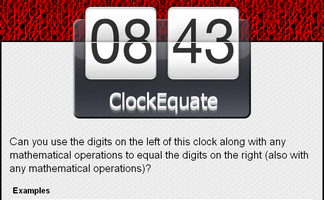Can you use the digits on the left of this clock along with any mathematical operations to equal the digits on the right?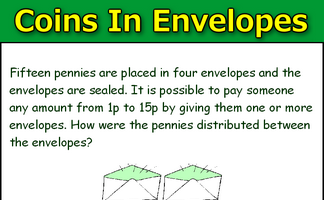Fifteen pennies are placed in four envelopes and the envelopes are sealed. It is possible to pay someone any amount from 1p to 15p by giving them one or more envelopes. How were the pennies distributed between the envelopes?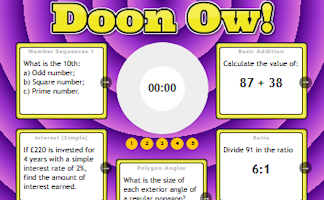What do you notice about the difference between the squares of consecutive numbers?Work out how many people were at the dance from the clues given.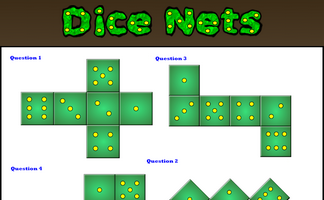Determine whether the given nets would fold to produce a dice.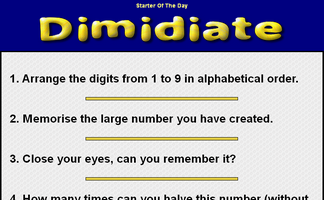Arrange the digits from 1 to 9 in alphabetical order. How many times can this number be halved?How old is a person if when her age is divided by certain numbers, the calculator display ending are as shown.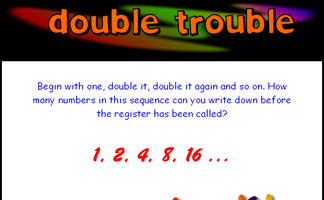Begin with one, double it, double it again and so on. How many numbers in this sequence can you write down before the register has been called?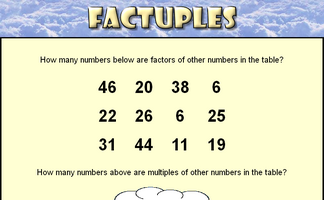Spot the factors and the multiples amongst the numbers in the grid.If each number in a sequence must be a factor or multiple of the previous number what is the longest sequence that can be made from the given numbers?Use the flowchart to generate a sequence of numbers. Which number will reach 1 the fastest?Find four single digit numbers that multiply together to give 120. How many different ways are there of answering this question?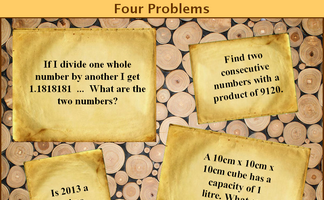For mathematical questions to get everyone thinking at the beginning of the lesson.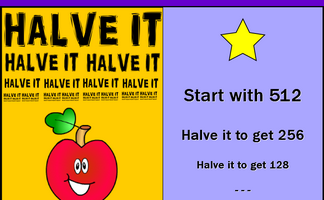Start with 512. Halve it to get 256. Halve it to get 128. Continue as far as possible.If all the students in this room shook hands with each other, how many handshakes would there be altogether?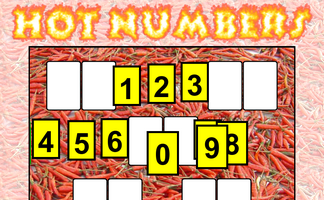Move the numbered cards to form five 2 digit numbers which are all multiples of three.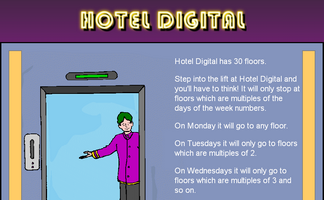A puzzle about the lifts in a hotel which serve floors based on the day of the week.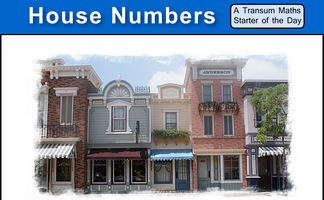The numbers on five houses next to each other add up to 70. What are those five numbers?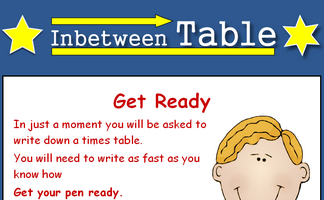Write down as many multiples of 3.5 as possible in 3.5 minutes.The 31st of December is the last day of the year. What mathematical lasts do you know?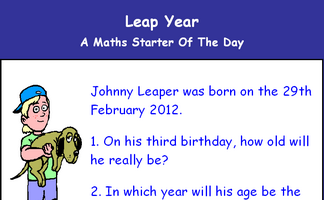A question about the birthdays of a child born on the 29th February.Questions about the number of letters in numbers.Which numbers when multiplied by the number of letters in the word(s) of the number give square numbers?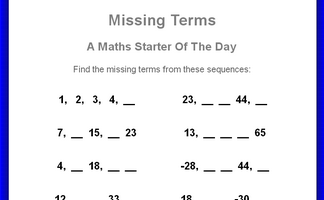Find the missing terms from these linear sequences.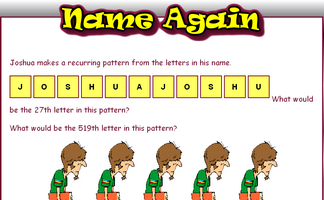Work out what the nth letter will be in a recurring pattern of letters in a person's namePerform calculations involving negative numbers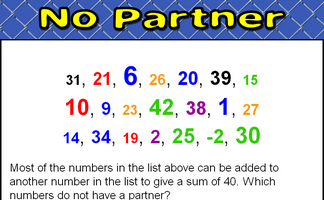Find which numbers in a given list do not combine with other numbers on the list to make a given sum.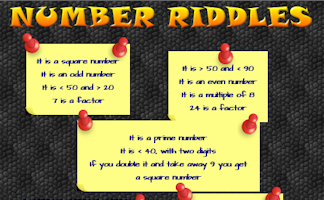Can you work out the numbers from the given clues.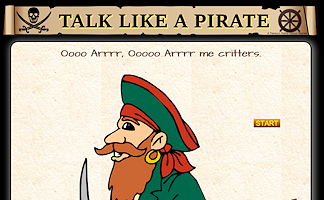Write out in words some numbers writen as digits (optional pirate theme)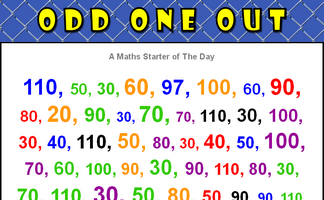From the numbers given, find the one that is the odd one out.Find other numbers that can be changed to 1 on a calculator using only the 4 key and any operation.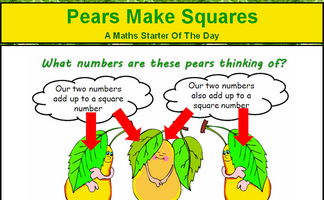Find three numbers such that each pair of numbers adds up to a square number.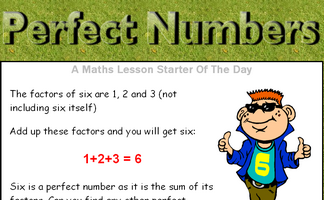Six is a perfect number as it is the sum of its factors. Can you find any other perfect numbers?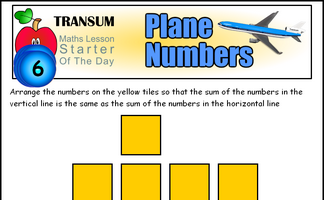Arrange numbers on the plane shaped grid to produce the given totalsArrange numbers at the bottom of the pyramid which will give the largest total at the top.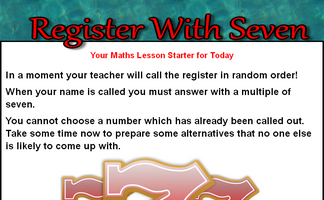When the register is called answer with a multiple of 7.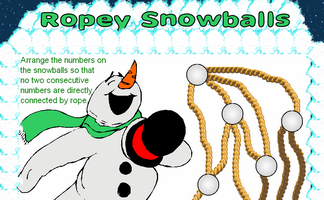Arrange the numbers on the snowballs so that no two consecutive numbers are directly connected by rope.Rearrange the numbers, row and column headings so that this table is mathematically correct.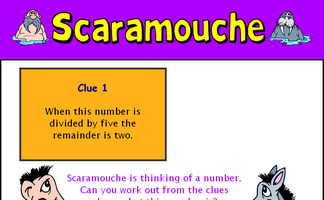Can you work out from the five clues given what the mystery number is?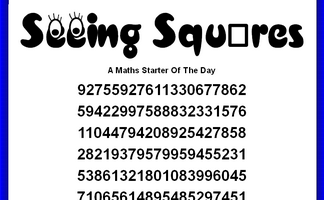How many square numbers can be found in the grid of digits.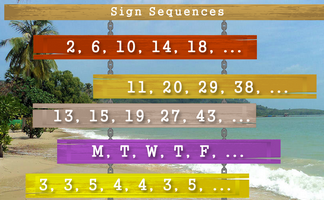Continue the sequences if you can work out the rule.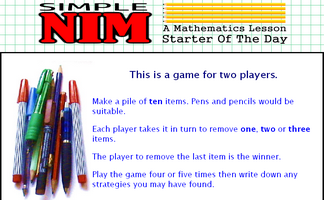The classic game of Nim played with a group of pens and pencils. The game can be extended to the multi-pile version.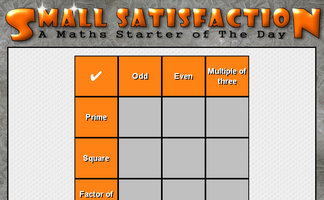Arrange the digits one to nine in the grid so that they obey the row and column headings.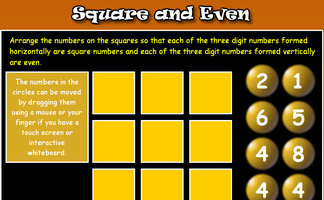Arrange the numbers on the cards so that each of the three digit numbers formed horizontally are square numbers and each of the three digit numbers formed vertically are even.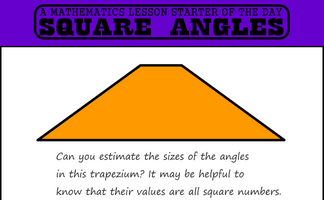Find a trapezium, a triangle and a quadrilateral where all of the angles are square numbers.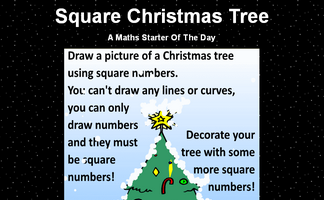Draw a picture of a Christmas tree using only square numbers.Arrange the numbered trees so that adjacent sums are square numbers.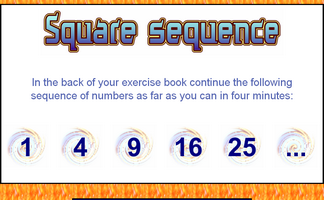Write out as many square numbers as possible in 4 minutes.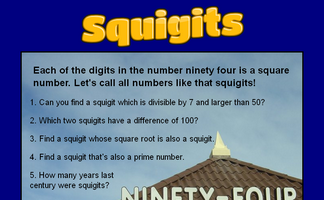A challenge to find numbers which have each of their digits as square numbers.Find a power of 2 and a power of 3 that are consecutive numbers.Be creative and come up with as many facts about a number as you can think of.How can you put the dice into the tins so that there is an odd number of dice in each tin?A Maths puzzle based on the 12 Days of Christmas song.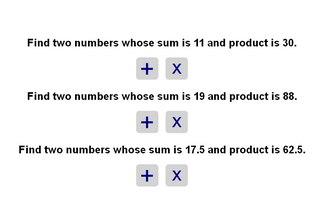Find the two numbers whose sum and product are given.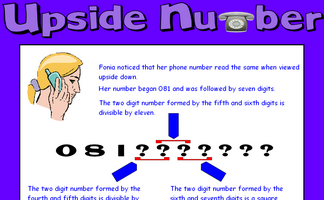Work out the phone number from the clues given.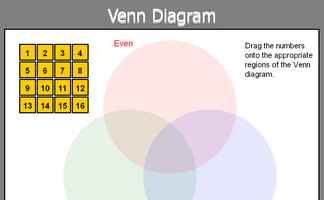Arrange numbers on the Venn Diagram according to their properties.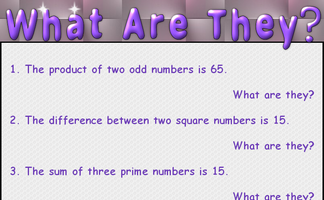A starter about sums, products, differences, ratios, square and prime numbers.

### Search

The activity you are looking for may have been classified in a different way from the way you were expecting. You can search the whole of Transum Maths by using the box below.

Have today's Starter of the Day as your default homepage. Copy the URL below then select
Tools > Internet Options (Internet Explorer) then paste the URL into the homepage field.

Set as your homepage (if you are using Internet Explorer)

Do you have any comments? It is always useful to receive feedback and helps make this free resource even more useful for those learning Mathematics anywhere in the world. Click here to enter your comments.For All: